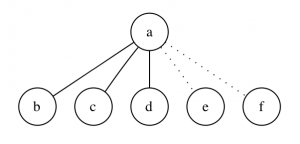## Six People at a Party

When there are six people at a party there will always be either:

1. At least three people who all know each other.
2. At least three people, none of whom know each other.

One of the above statements must be true. You can’t have a party of six people where everybody knows one other person but there’s no group of three people none of whom know each other, for example.

This can be shown using graph theory.

1. We represent the group of six people with a graph.
2. Edges between vertices are of two types (know or don’t know).
3. Pick a vertex at random. Any vertex will have at least 3 edges of one type. For example:Where known/not known is represented by solid/dotted.

4. Now, either one of the edges bc, bd or cd is solid or not.

5. If one edge is solid, 3 people all know/don’t know each other (abc,acd, or abd).

6. If none of the edges exists then bcd know/don’t know each other.

## Notes

The dot file used to generate the graph above:

```graph graphname {
a [shape=circle];
b [shape=circle];
c [shape=circle];
d [shape=circle];
e [shape=circle];
f [shape=circle];

a -- b;
a -- c;
a -- d;
a -- e [style=dotted];
a -- f [style=dotted];
}
```

I encountered this problem in “Introduction to Graph Theory” by Robin J. Wilson (great book).

You can read more about the problem and it’s generalisation on wikipedia here.